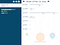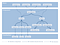# On D3, React, and a little bit of FluxThe example expense app

# D3 and React

## Entering and Exiting with React

`var graph = d3.select(‘svg’).append(‘g’) .classed(‘graph’, true);var expenses = graph.selectAll(‘g.expense’);var entered = expenses .data(expensesData, (expense) => expense.id) // use id as key .enter().append(‘g’) .classed(‘expense’, true);entered.append(‘rect’) .classed(‘expenseRect’, true);entered.append(‘rect’) .classed(‘textBG’, true);entered.append(‘text’);expenses.exit().remove();`
`class ExpenseComponent extends React.Component { render() {  return (   <g className=”expense”>    <rect className=”expenseRect” />    <rect className=”textBG” />    <text />   </g>  ); }}class GraphComponent extends React.Component { render() {  var expenses = expensesData && expensesData.map((expense) => {    // go through all data and return components keyed by id     return (<ExpenseComponent key={expense.id} data={expense} />);  });  return (   <svg>    <g className=”graph”>     {expenses}    </g>   </svg> );}`
`entered.select(‘rect’) .classed(‘textBG’, true) .classed(‘hidden’, (d) => !d.name);entered.select(‘text’) .classed(‘hidden’, (d) => !d.name);`
`class ExpenseComponent extends React.Component { render() {  return (   <g className=”expense”>    <rect className=”expenseRect” />    {this.props.data.name && (<rect className=”textBG” />)}    {this.props.data.name && (<text />)}   </g>  ); }}`

## Updating and Transitioning with D3

`var ExpenseVisualization = {};ExpenseVisualization.enter = (selection) => { selection.select(‘rect.expenseRect’)  .attr(‘x’, (d) => -d.size / 2)  .attr(‘y’, (d) => -d.size / 2)  // …  .attr(‘stroke-width’, 0); selection.select(‘rect.textBG’)  .attr(‘opacity’, 0)  // …  .attr(‘fill’, ‘#fafafa’); selection.select(‘text’)  // …  .attr(‘opacity’, 0)  .text((d) => d.name);  selection  .attr(‘transform’, (d) => ‘translate(‘ + d.x + ‘,’ + d.y + ‘)’);}ExpenseVisualization.update = (selection) => { selection.select(‘rect.expenseRect’)  .transition().duration(duration)  .attr(‘width’, (d) => d.size)  .attr(‘height’, (d) => d.size)  // … animate box in; selection.select(‘text’)  // … position text element; selection.select(‘rect.textBG’)  // … position text background; selection  .transition().duration(duration)  .attr(‘transform’, (d) => ‘translate(‘ + d.x + ‘,’ + d.y + ‘)’);}`
`class ExpenseComponent extends React.Component { componentDidMount() {  // wrap element in d3  this.d3Node = d3.select(this.getDOMNode());  this.d3Node.datum(this.props.data)   .call(ExpenseVisualization.enter); }, shouldComponentUpdate(nextProps) {  if (nextProps.data.update) {   // use d3 to update component   this.d3Node.datum(nextProps.data)    .call(ExpenseVisualization.update);   return false;  }  return true; }, componentDidUpate() {  this.d3Node.datum(this.props.data)   .call(ExpenseVisualization.update); }, render() {  // … }}`

## More on transitioning with D3

`ExpenseVisualization.update = (selection) => { selection  .transition().delay((d, i) => d.order * duration)  .duration(duration)  .attr(‘transform’, (d) => ‘translate(‘ + d.x + ‘,’ + d.y + ‘)’);}GraphVisualization.update = (selection) => { selection.selectAll(‘.expense’)  .call(ExpenseVisualization.update);}class GraphComponent extends React.Component { componentDidMount() {  this.d3Node = d3.select(this.getDOMNode()); }, componentDidUpdate() {  this.d3Node.call(GraphVisualization.update); }}`

# A little bit of FluxHow the code is structured
`class GraphComponent extends React.Component { getInitialState() {  return {   categories: [],   expenses: [],   links: []  } }, componentDidMount() {  GraphStore.addChangeListener(this._onChange);   SelectionStore.addChangeListener(this._onChange); }, componentWillReceiveProps(nextProps) {  this._onChange(nextProps); }, componentWillUnMount() {  GraphStore.removeChangeListener(this._onChange);  SelectionStore.removeChangeListener(this._onChange); }, // use the next props to calculate and set the next state _onChange(props, width, height) {  props = props || this.props;  var selection = SelectionStore.getSelection();  var categories = AppCalculationUtils.calculateCategories(props.data.expenses);  var expenses = AppCalculationUtils.calculateExpenses(props.data.expenses);  var links = AppCalculationUtils.calculateLinks(categories, expenses);  // calculate some more rendering things  AppCalculationUtils.calculateSizes(categories);  AppCalculationUtils.highlightSelections(selection, categories, expenses);  // calculate their positions  AppCalculationUtils.positionExpenses(expenses);  AppCalculationUtils.positionGraph(categories, expenses, links);  var state = {categories, expenses, links, dates, width, height};  AppCalculationUtils.calculateUpdate(this.state, state);  this.setState(state); }, render() {  var links = this.state.links.map((link) => {   var key = link.source.id + ‘,’ + link.target.id;   return (<LinkComponent key={key} data={link} />);  });  var categories = this.state.categories.map((category) => {   return (<CategoryComponent key={category.id} data={category} />);  });  var expenses = this.state.expenses.map((expense) => {   return (<ExpenseComponent key={expense.id} data={expense} />);  });  return (   <svg>    <g className=”graph”>     {links}     {categories}     {expenses}    </g>   </svg>  ); }}`

## A note on updating

`AppCalculationUtils.calculateUpdate = (prev, next) => { _.each(next.expenses, (expense) => {  var prevExpense = _.find(prev.expenses, (prevExpense) => prevExpense.id === expense.id);  if (prevExpense) {   delete prevExpense.update;   expense.update = !_.isEqual(prevExpense, expense);  } }); // do the same with categories and links}`
`class ExpenseComponent extends React.Component { shouldComponentUpdate(nextProps) {  if (nextProps.data.update) {   this.d3Node.datum(nextProps.data)    .call(CategoryVisualization.update);   return false;  }  return true; }}`

# In Conclusion

Written by

## Shirley Wu

#### I code too much & don’t draw enough. @ucberkeley alum, 1/2 @datasketches, @d3bayarea & @d3unconf co-organizer ✨ currently freelancing → http://sxywu.com

Welcome to a place where words matter. On Medium, smart voices and original ideas take center stage - with no ads in sight. Watch
Follow all the topics you care about, and we’ll deliver the best stories for you to your homepage and inbox. Explore
Get unlimited access to the best stories on Medium — and support writers while you’re at it. Just \$5/month. Upgrade